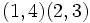# Element structure of Klein four-group

Jump to: navigation, search

## Contents

This article gives specific information, namely, element structure, about a particular group, namely: Klein four-group.
View element structure of particular groups | View other specific information about Klein four-group

The Klein four-group is the unique (up to isomorphism) non-cyclic group of order four. In this group, every non-identity element has order two.

The multiplication table with non-identity elements$a,b,c$ and identity element$e$:

Element/element$e$$a$$b$$c$$e$$e$$a$$b$$c$$a$$a$$e$$c$$b$$b$$b$$c$$e$$a$$c$$c$$b$$a$$e$

The multiplication table can be described as follows (and this characterizes the group):

• The product of the identity element and any element is that element itself.
• The product of any non-identity element with itself is the identity element.
• The product of two distinct non-identity elements is the third non-identity element.

## Elements

Below is a description of the elements for the many alternate descriptions of the Klein four-group. Note that the choice of correspondence between the descriptions is somewhat arbitrary, in the sense that it can be modified by automorphisms of the Klein four-group, which include arbitrary permutations of the three non-identity elements (see endomorphism structure of Klein four-group).

Element Interpretation as$\mathbb{Z}/2\mathbb{Z} \times \mathbb{Z}/2\mathbb{Z}$, viewing$\mathbb{Z}/2\mathbb{Z}$ as the additive group of integers mod 2 and$\times$ denotes external direct product Interperation as$\{ \pm 1 \} \times \{ \pm 1 \}$ Interpretation as double transposition$e$ (0,0) (1,1)$()$$a$ (1,0) (-1,1)$(1,2)(3,4)$$b$ (0,1) (1,-1)$(1,3)(2,4)$$c$ (1,1) (-1,-1)$(1,4)(2,3)$

Here is the multiplication table (better termed an addition table, since we are carrying out coordinate-wise addition mod 2) viewed as a direct product of two copies of the additive group of integers mod 2:

Element/element (0,0) (1,0) (0,1) (1,1)
(0,0) (0,0) (1,0) (0,1) (1,1)
(1,0) (1,0) (0,0) (1,1) (0,1)
(0,1) (0,1) (1,1) (0,0) (1,0)
(1,1) (1,1) (0,1) (1,0) (0,0)

Here is the multiplication table viewed as a direct product of two copies of$\{ -1, 1 \}$:

Element/element (1,1) (-1,1) (1,-1) (-1,-1)
(1,1) (1,1) (-1,1) (1,-1) (-1,-1)
(-1,1) (-1,1) (1,1) (-1,-1) (1,-1)
(1,-1) (1,-1) (-1,-1) (1,1) (-1,1)
(-1,-1) (-1,-1) (1,-1) (-1,1) (1,1)